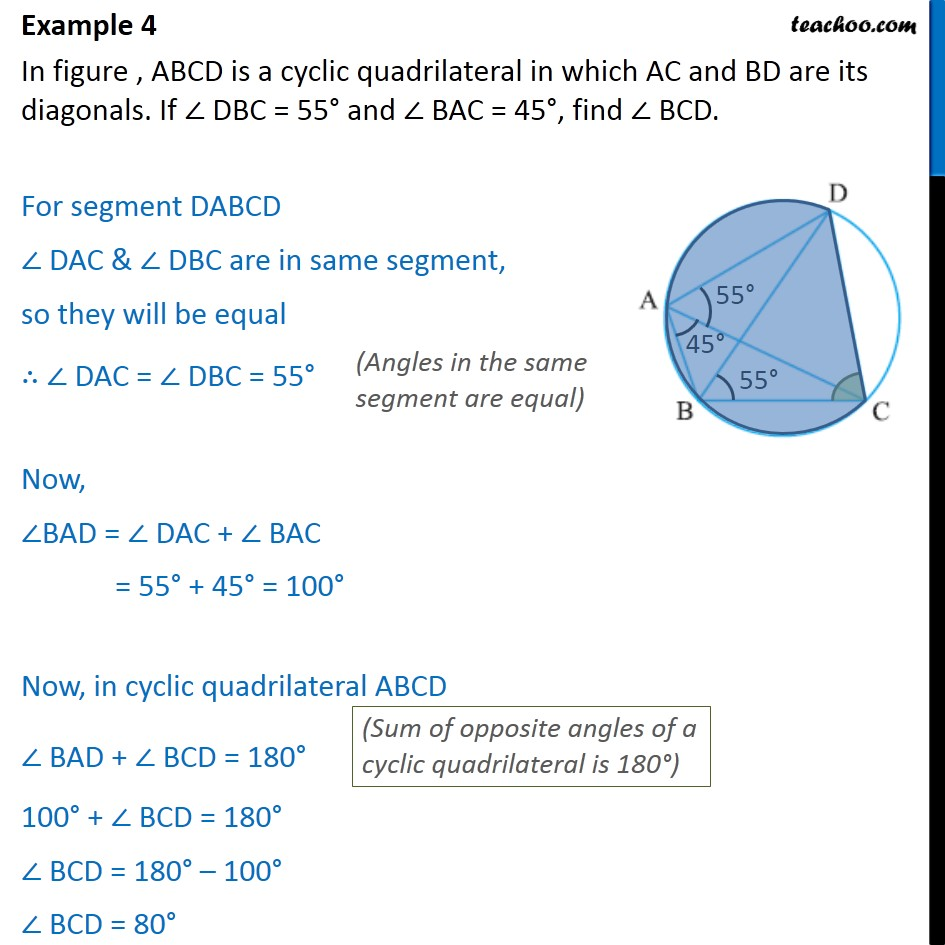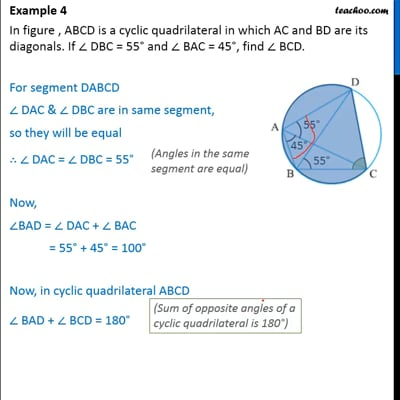Examples

Chapter 10 Class 9 Circles (Term 2)
Serial order wiseThis video is only available for Teachoo black users

### Transcript

Example 4 In figure , ABCD is a cyclic quadrilateral in which AC and BD are its diagonals. If DBC = 55 and BAC = 45 , find BCD. For segment DABCD DAC & DBC are in same segment, so they will be equal DAC = DBC = 55 Now, BAD = DAC + BAC = 55 + 45 = 100 Now, in cyclic quadrilateral ABCD BAD + BCD = 180 100 + BCD = 180 BCD = 180 100 BCD = 80